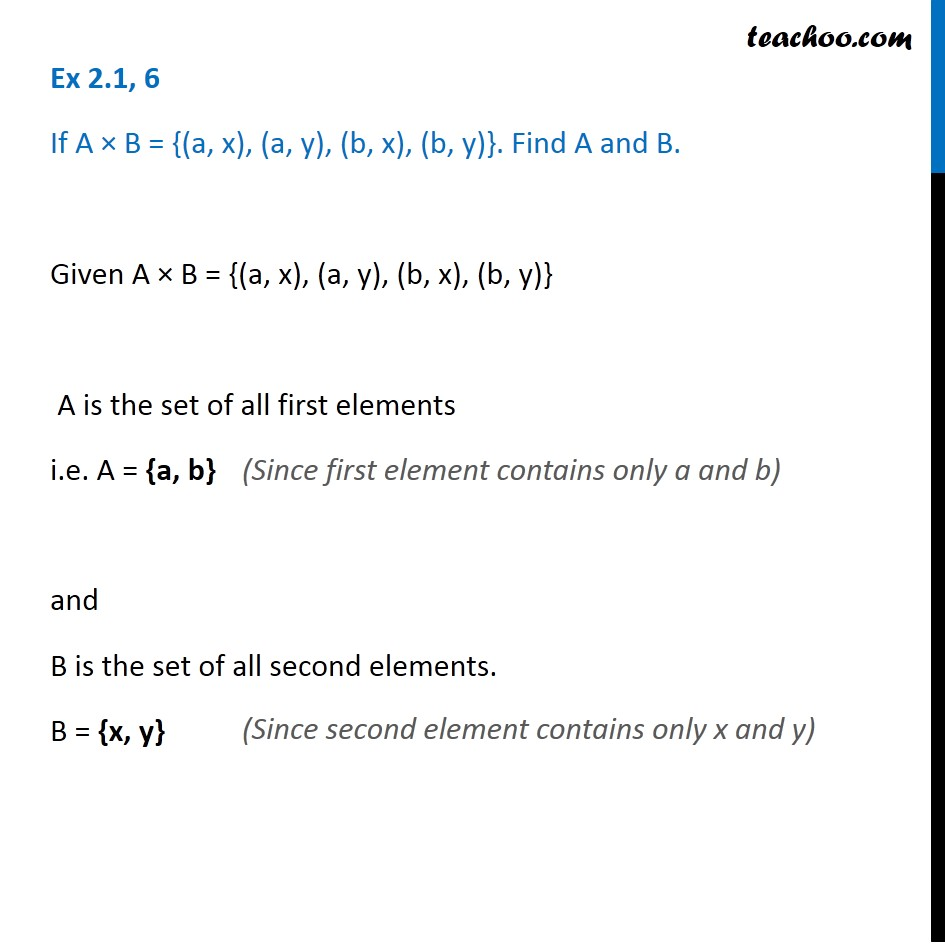Ex 2.1

Chapter 2 Class 11 Relations and Functions
Serial order wiseLearn in your speed, with individual attention - Teachoo Maths 1-on-1 Class

### Transcript

Ex 2.1, 6 If A × B = {(a, x), (a, y), (b, x), (b, y)}. Find A and B. Given A × B = {(a, x), (a, y), (b, x), (b, y)} A is the set of all first elements i.e. A = {a, b} and B is the set of all second elements. B = {x, y} (Since first element contains only a and b) (Since second element contains only x and y)